# The g-h Filter¶

In :
%matplotlib inline

In :
#format the book
import book_format
book_format.set_style()

Out:

Before we start, be sure you understand how to use Jupyter Notebooks, and are familiar with the SciPy, NumPy, and Matplotlib packages, as they are used throughout this book. The Preface contains an introduction to these packages.

## Building Intuition via Thought Experiments¶

Imagine that we live in a world without scales - the devices you stand on to weigh yourself. One day at work a co-worker comes running up to you and announces her invention of a 'scale' to you. After she explains, you eagerly stand on it and announce the results: "172 lbs". You are ecstatic - for the first time in your life you know what you weigh. More importantly, dollar signs dance in your eyes as you imagine selling this device to weight loss clinics across the world! This is fantastic!

Another co-worker hears the commotion and comes over to find out what has you so excited. You explain the invention and once again step onto the scale, and proudly proclaim the result: "161 lbs." And then you hesitate, confused.

"It read 172 lbs a few seconds ago", you complain to your co-worker.

"I never said it was accurate," she replies.

Sensors are inaccurate. This is the motivation behind a huge body of work in filtering, and solving this problem is the topic of this book. I could just provide the solutions that have been developed over the last half century, but these solutions were developed by asking very basic, fundamental questions into the nature of what we know and how we know it. Before we attempt the math, let's follow that journey of discovery, and see if it informs our intuition about filtering.

Try Another Scale

Is there any way we can improve upon this result? The obvious, first thing to try is get a better sensor. Unfortunately, your co-worker informs you that she has built 10 scales, and they all operate with about the same accuracy. You have her bring out another scale, and you weigh yourself on one, and then on the other. The first scale (A) reads "160 lbs", and the second (B) reads "170 lbs". What can we conclude about your weight?

Well, what are our choices?

• We could choose to only believe A, and assign 160lbs to our weight estimate.
• We could choose to only believe B, and assign 170lbs to our weight.
• We could choose a number less than both A and B.
• We could choose a number greater than both A and B.
• We could choose a number between A and B.

The first two choices are plausible, but we have no reason to favor one scale over the other. Why would we choose to believe A instead of B? We have no reason for such a belief. The third and fourth choices are irrational. The scales are admittedly not very accurate, but there is no reason at all to choose a number outside of the range of what they both measured. The final choice is the only reasonable one. If both scales are inaccurate, and as likely to give a result above my actual weight as below it, more often than not the answer is somewhere between A and B.

In mathematics this concept is formalized as expected value, and we will cover it in depth later. For now ask yourself what would be the 'usual' thing to happen if we took one million readings. Some of the times both scales will read too low, sometimes both will read too high, and the rest of the time they will straddle the actual weight. If they straddle the actual weight then certainly we should choose a number between A and B. If they don't straddle then we don't know if they are both too high or low, but by choosing a number between A and B we at least mitigate the effect of the worst measurement. For example, suppose our actual weight is 180 lbs. 160 lbs is a big error. But if we choose a weight between 160 lbs and 170 lbs our estimate will be better than 160 lbs. The same argument holds if both scales returned a value greater than the actual weight.

We will deal with this more formally later, but for now I hope it is clear that our best estimate is the average of A and B.

$$\frac{160+170}{2} = 165$$

We can look at this graphically. I have plotted the measurements of A and B with an assumed error of $\pm$ 8 lbs. The measurements falls between 160 and 170 so the only weight that makes sense must lie within 160 and 170 lbs.

In :
import kf_book.book_plots as book_plots
from kf_book.book_plots import plot_errorbars
plot_errorbars([(160, 8, 'A'), (170, 8, 'B')], xlims=(150, 180))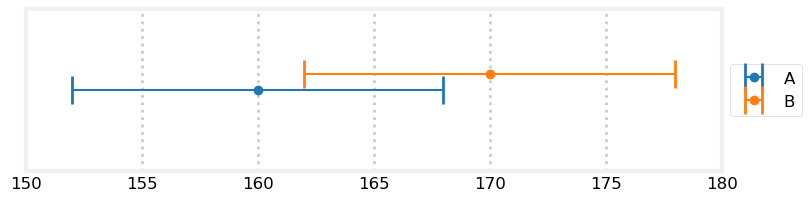A word on how I generated this plot. I import code from the module book_plots in the kf_book subdirectory. Generating this plot takes a lot of boilerplate Python that isn't interesting to read. I take this tack often in the book. When the cell is run plot_errorbars() gets called and the plot is inserted into the book.

If this is your first time using Jupyter Notebook, the code above is in a cell. The text "In :" labels this as a cell where you can enter input, and the number in the bracket denotes that this cell was run second. To run the cell, click on it with your mouse so that it has focus, then press CTRL+ENTER on the keyboard. As we continue you will be able to alter the code inside the cells and rerun them. Try changing the values "160", "170", and "8" to some other value and run the cell. The printed output should change depending on what you entered.

If you want to view the code for plot_errorbars, either open it in an editor, or create a new cell and type the function name followed by two question marks. Press Ctrl+Enter, and your browser will open a window displaying the source code. This is a feature of Jupyter Notebooks. If you want to just view the documentation for the function, do the same but with one question mark.

plot_errorbars??


or

plot_errorbars?


So 165 lbs looks like a reasonable estimate, but there is more information here that we might be able to take advantage of. The only weights that are possible lie in the intersection between the error bars of A and B. For example, a weight of 161 lbs is impossible because scale B could not give a reading of 170 lbs with a maximum error of 8 pounds. Likewise a weight of 169 lbs is impossible because scale A could not give a reading of 160 lbs with a maximum error of 8 lbs. In this example the only possible weights lie in the range of 162 to 168 lbs.

That doesn't yet allow us to find a better weight estimate, but let's play 'what if' some more. What if we are now told that A is three times more accurate than B? Consider the 5 options we listed above. It still makes no sense to choose a number outside the range of A and B, so we will not consider those. It perhaps seems more compelling to choose A as our estimate - after all, we know it is more accurate, why not use it instead of B? Can B possibly improve our knowledge over A alone?

The answer, perhaps counter intuitively, is yes, it can. First, let's look at the same measurements of A=160 and B=170, but with the error of A $\pm$ 3 lbs and the error of B is 3 times as much, $\pm$ 9 lbs.

In :
plot_errorbars([(160, 3, 'A'), (170, 9, 'B')], xlims=(150, 180))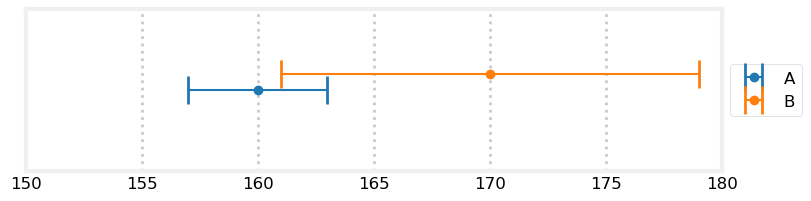The overlap of the error bars of A and B are the only possible true weight. This overlap is smaller than the error in A alone. More importantly, in this case we can see that the overlap doesn't include 160 lbs or 165 lbs. If we only used the measurement from A because it is more accurate than B we would give an estimate of 160 lbs. If we average A and B we would get 165 lbs. Neither of those weights are possible given our knowledge of the accuracy of the scales. By including the measurement of B we would give an estimate somewhere between 161 lbs and 163 lbs, the limits of the intersections of the two error bars.

Let's take this to the extreme limits. Assume we know scale A is accurate to 1 lb. In other words, if we truly weigh 170 lbs, it could report 169, 170, or 171 lbs. We also know that scale B is accurate to 9 lbs. We do a weighing on each scale, and get A=160, and B=170. What should we estimate our weight to be? Let's look at that graphically.

In :
plot_errorbars([(160, 1, 'A'), (170, 9, 'B')], xlims=(150, 180))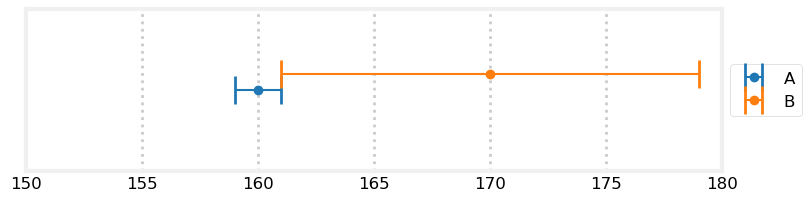Here we can see that the only possible weight is 161 lbs. This is an important result. With two relatively inaccurate sensors we are able to deduce an extremely accurate result.

So two sensors, even if one is less accurate than the other, is better than one. I will harp on this for the remainder of the book. We never throw information away, no matter how poor it is. We will be developing math and algorithms that allow us to include all possible sources of information to form the best estimate possible.

However, we have strayed from our problem. No customer is going to want to buy multiple scales, and besides, we initially started with an assumption that all scales were equally (in)accurate. This insight of using all measurements regardless of accuracy will play a large role later, so don't forget it.

What if I have one scale, but I weigh myself many times? We concluded that if we had two scales of equal accuracy we should average the results of their measurements. What if I weigh myself 10,000 times with one scale? We have already stated that the scale is equally likely to return a number too large as it is to return one that is too small. It is not that hard to prove that the average of a large number of weights will be very close to the actual weight, but let's write a simulation for now. I will use NumPy, part of the SciPy ecosystem for numerical computation.

In :
import numpy as np
measurements = np.random.uniform(160, 170, size=10000)
mean = measurements.mean()
print(f'Average of measurements is {mean:.4f}')

Average of measurements is 164.9683


The exact number printed depends on your random number generator, but it should be very close to 165.

This code makes one assumption that probably isn't true - that the scale is as likely to read 160 as 165 for a true weight of 165 lbs. This is almost never true. Real sensors are more likely to get readings nearer the true value, and are less and less likely to get readings the further away from the true value it gets. We will cover this in detail in the Gaussian chapter. For now, I will use without further explanation the numpy.random.normal() function, which will produce more values nearer 165 lbs, and fewer further away. Take it on faith for now that this will produce noisy measurements similar to how a real scale works.

In :
mean = np.random.normal(165, 5, size=10000).mean()
print(f'Average of measurements is {mean:.4f}')

Average of measurements is 164.9450


Again the answer is very close to 165.

Okay, great, we have an answer to our sensor problem! But it is not a very practical answer. No one has the patience to weigh themselves ten thousand, or even a dozen times.

So, let's play 'what if'. What if you measured your weight once a day, and got the readings 170, 161, and then 169. Did you gain weight, lose weight, or is this all just noisy measurements?

We really can't say. The first measurement was 170, and the last was 169, implying a 1 lb loss. But if the scale is only accurate to 10 lbs, that is explainable by noise. I could have actually gained weight; maybe my weight on day one was 165 lbs, and on day three it was 172. It is possible to get those weight readings with that weight gain. My scale tells me I am losing weight, and I am actually gaining weight! Let's look at that in a chart. I've plotted the measurements along with the error bars, and then some possible weight gain/losses that could be explained by those measurements in dotted green lines.

In :
import kf_book.gh_internal as gh
gh.plot_hypothesis1()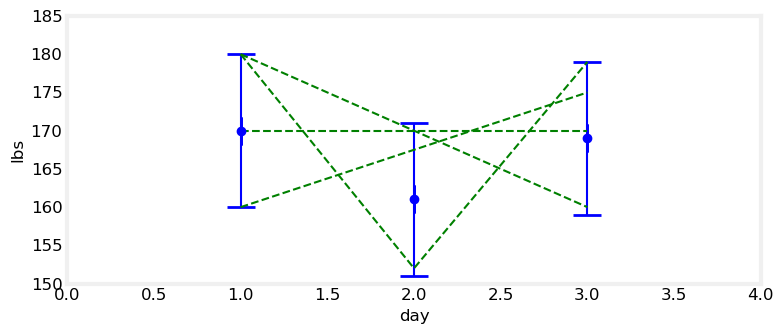As we can see there is an extreme range of weight changes that could be explained by these three measurements. In fact, there are an infinite number of choices. Shall we give up? Not me! Recall that we are talking about measuring a human's weight. There is no reasonable way for a human to weigh 180 lbs on day 1 and 160 lbs on day 3. or to lose 30 lbs in one day only to gain it back the next (we will assume no amputations or other trauma has happened to the person).

The behavior of the physical system we are measuring should influence how we interpret the measurements. If we were weighing a rock each day we'd attribute all of the variance to noise. If we were weighing a cistern fed by rain and used for household chores we might believe such weight changes are real.

Suppose I take a different scale, and I get the following measurements: 169, 170, 169, 171, 170, 171, 169, 170, 169, 170. What does your intuition tell you? It is possible, for example, that you gained 1 lb each day, and the noisy measurements just happens to look like you stayed the same weight. Equally, you could have lost 1 lb a day and gotten the same readings. But is that likely? How likely is it to flip a coin and get 10 heads in a row? Not very likely. We can't prove it based solely on these readings, but it seems pretty likely that my weight held steady. In the chart below I've plotted the measurements with error bars, and a likely true weight in dashed green. This dashed line is not meant to be the 'correct' answer to this problem, merely one that is reasonable and could be explained by the measurement.

In :
gh.plot_hypothesis2()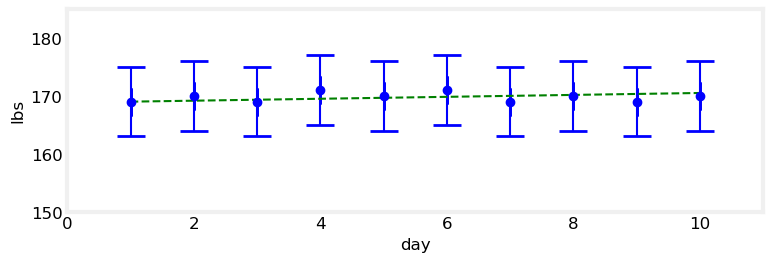Another what if: what if the readings were 158.0, 164.2, 160.3, 159.9, 162.1, 164.6, 169.6, 167.4, 166.4, 171.0? Let's look at a chart of that and then answer some questions.

In :
gh.plot_hypothesis3()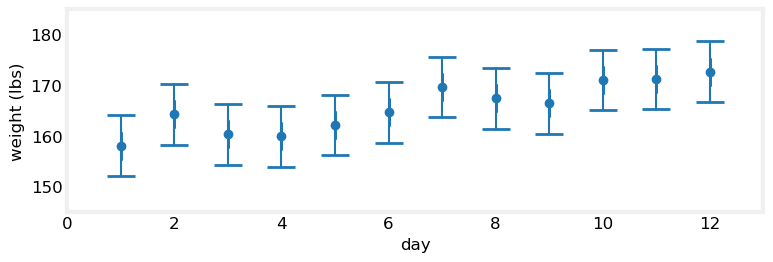Does it 'seem' likely that I lost weight and this is just really noisy data? Not really. Does it seem likely that I held the same weight? Again, no. This data trends upwards over time; not evenly, but definitely upwards. We can't be sure, but that looks like a weight gain, and a significant weight gain at that. Let's test this assumption with some more plots. It is often easier to 'eyeball' data in a chart versus a table.

So let's look at two hypotheses. First, let's assume our weight did not change. To get that number we agreed that we should average the measurements. Let's look at that.

In :
gh.plot_hypothesis4()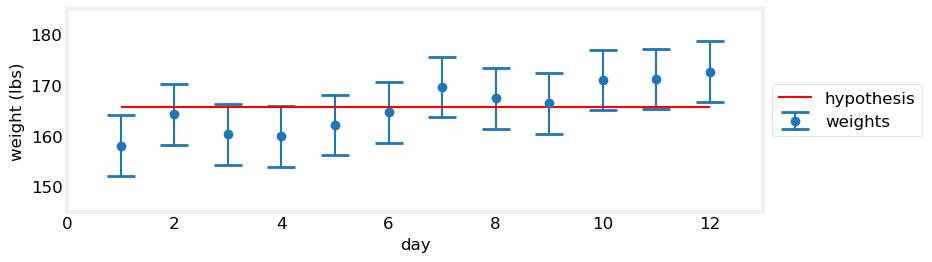That doesn't look very convincing. In fact, we can see that there is no horizontal line that we could draw that is inside all of the error bars.

Now, let's assume we gained weight. How much? I don't know, but NumPy does! We want to draw a line through the measurements that looks 'about' right. NumPy has functions that will do this according to a rule called "least squares fit". Let's not worry about the details of that computation (I use polyfit() if you are interested), and just plot the results.

In :
gh.plot_hypothesis5()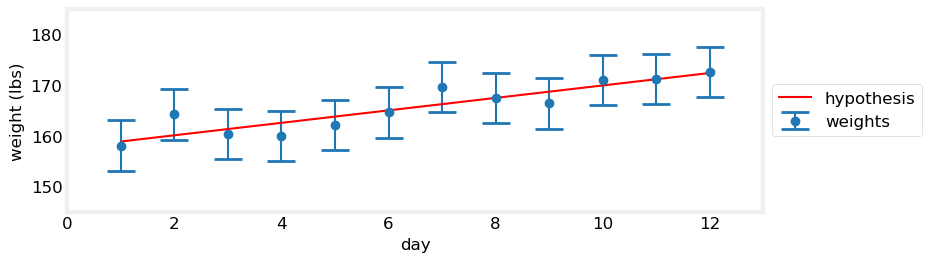This looks much better, at least to my eyes. Notice now the hypothesis lies very close to each measurement, whereas in the previous plot the hypothesis was often quite far from the measurement. It seems far more likely to be true that I gained weight than I didn't gain any weight. Did I actually gain 13 lbs? Who can say? That seems impossible to answer.

"But is it impossible?" pipes up a co-worker.

Let's try something crazy. Let's assume that I know I am gaining about one lb a day. It doesn't matter how I know that right now, assume I know it is approximately correct. Maybe I am on a 6000 calorie a day diet, which would result in such a weight gain. Or maybe there is another way to estimate the weight gain. This is a thought experiment, the details are not important. Let's see if we can make use of such information if it was available.

The first measurement was 158. We have no way of knowing any different, so let's accept that as our estimate. If our weight today is 158, what will it be tomorrow? Well, we think we are gaining weight at 1 lb/day, so our prediction is 159, like so:

In :
gh.plot_estimate_chart_1()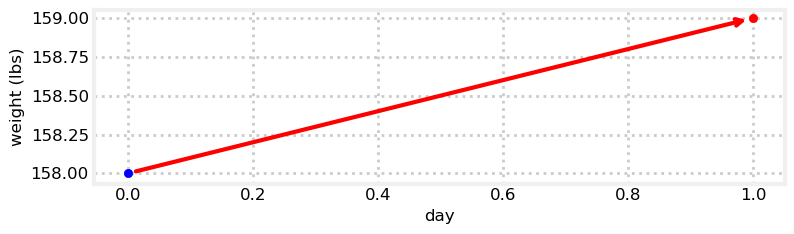Okay, but what good is this? Sure, we could assume the 1 lb/day is accurate, and predict our weight for the next 10 days, but then why use a scale at all if we don't incorporate its readings? So let's look at the next measurement. We step on the scale again and it displays 164.2 lbs.

In :
gh.plot_estimate_chart_2()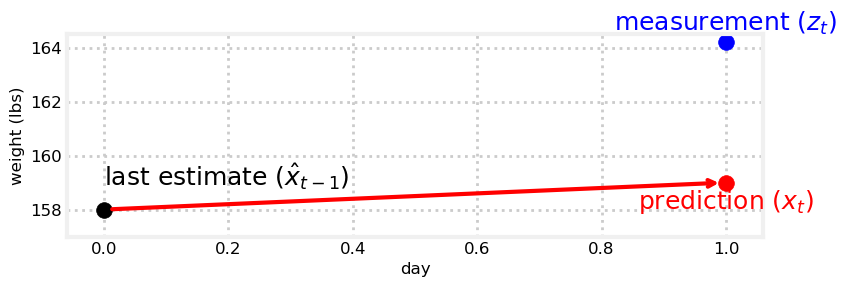We have a problem. Our prediction doesn't match our measurement. But, that is what we expected, right? If the prediction was always exactly the same as the measurement, it would not be capable of adding any information to the filter. And, of course, there would be no reason to ever measure since our predictions are perfect.

The key insight to this entire book is in the next paragraph. Read it carefully!

So what do we do? If we only form estimates from the measurement then the prediction will not affect the result. If we only form estimates from the prediction then the measurement will be ignored. If this is to work we need to take some kind of blend of the prediction and measurement (I've bolded the key point).

Blending two values - this sounds a lot like the two scale problem earlier. Using the same reasoning as before we can see that the only thing that makes sense is to choose a number between the prediction and the measurement. For example, an estimate of 165 makes no sense, nor does 157. Our estimates should lie between 159 (the prediction) and 164.2 (the measurement).

One more time, this is so important. We agreed that when presented two values with errors, we should form an estimate part way between the two values. It does not matter how those values were generated. In the start of the chapter we had two measurements, but now we have one measurement and one prediction. The reasoning, and hence the math is the same in both cases. We never throw information away. I mean it. I see so much commercial software that throws away noisy data. Don't do it! Our prediction of a weight gain might not be very accurate, but so long as there is some information we should use it.

I have to insist you stop and really think about this. All I have done is replaced an inaccurate scale with an inaccurate weight prediction based on human physiology. It is still data. Math doesn't know if the data came from a scale or a prediction. We have two pieces of data with a certain amount of noise, and we want to combine them. In the remainder of this book we are going to develop some fairly complicated math to perform this computation, but the math never cares where the data come from, it only makes computations based on the value and accuracy of those values.

Should the estimate be half way between the measurement and prediction? Maybe, but in general it seems like we might know that our prediction is more or less accurate compared to the measurements. Probably the accuracy of our prediction differs from the accuracy of the scale. Recall what we did when scale A was much more accurate than scale B - we scaled the answer to be closer to A than B. Let's look at that in a chart.

In :
gh.plot_estimate_chart_3()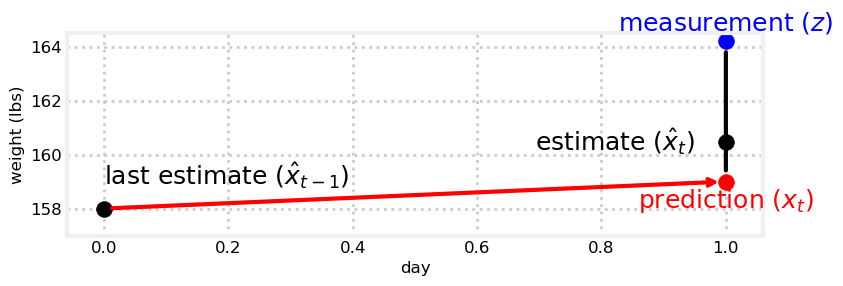Now let's try a randomly chosen number to scale our estimate: $\frac{4}{10}$. Our estimate will be four tenths the measurement and the rest will be from the prediction. In other words, we are expressing a belief here, a belief that the prediction is somewhat more likely to be correct than the measurement. We compute that as

$$\mathtt{estimate} = \mathtt{prediction} + \frac{4}{10}(\mathtt{measurement} - \mathtt{prediction})$$

The difference between the measurement and prediction is called the residual, which is depicted by the black vertical line in the plot above. This will become an important value to use later on, as it is an exact computation of the difference between measurements and the filter's output. Smaller residuals imply better performance.

Let's code that and see the results when we test it against the series of weights from above. We have to take into account one other factor. Weight gain has units of lbs/time, so to be general we will need to add a time step $t$, which we will set to 1 (day).

I hand generated the weight data to correspond to a true starting weight of 160 lbs, and a weight gain of 1 lb per day. In other words on the first day (day zero) the true weight is 160lbs, on the second day (day one, the first day of weighing) the true weight is 161 lbs, and so on.

We need to make a guess for the initial weight. It is too early to talk about initialization strategies, so for now I will assume 160 lbs.

In :
from kf_book.book_plots import figsize
import matplotlib.pyplot as plt

weights = [158.0, 164.2, 160.3, 159.9, 162.1, 164.6,
169.6, 167.4, 166.4, 171.0, 171.2, 172.6]

time_step = 1.0  # day
scale_factor = 4.0/10

def predict_using_gain_guess(estimated_weight, gain_rate, do_print=False):
# storage for the filtered results
estimates, predictions = [estimated_weight], []

# most filter literature uses 'z' for measurements
for z in weights:
# predict new position
predicted_weight = estimated_weight + gain_rate * time_step

# update filter
estimated_weight = predicted_weight + scale_factor * (z - predicted_weight)

# save and log
estimates.append(estimated_weight)
predictions.append(predicted_weight)
if do_print:
gh.print_results(estimates, predicted_weight, estimated_weight)

return estimates, predictions

initial_estimate = 160.
estimates, predictions = predict_using_gain_guess(
estimated_weight=initial_estimate, gain_rate=1, do_print=True)

previous estimate: 160.00, prediction: 161.00, estimate 159.80
previous estimate: 159.80, prediction: 160.80, estimate 162.16
previous estimate: 162.16, prediction: 163.16, estimate 162.02
previous estimate: 162.02, prediction: 163.02, estimate 161.77
previous estimate: 161.77, prediction: 162.77, estimate 162.50
previous estimate: 162.50, prediction: 163.50, estimate 163.94
previous estimate: 163.94, prediction: 164.94, estimate 166.80
previous estimate: 166.80, prediction: 167.80, estimate 167.64
previous estimate: 167.64, prediction: 168.64, estimate 167.75
previous estimate: 167.75, prediction: 168.75, estimate 169.65
previous estimate: 169.65, prediction: 170.65, estimate 170.87
previous estimate: 170.87, prediction: 171.87, estimate 172.16

In :
# plot results
book_plots.set_figsize(10)
gh.plot_gh_results(weights, estimates, predictions, [160, 172])
weights

Out:
[158.0,
164.2,
160.3,
159.9,
162.1,
164.6,
169.6,
167.4,
166.4,
171.0,
171.2,
172.6]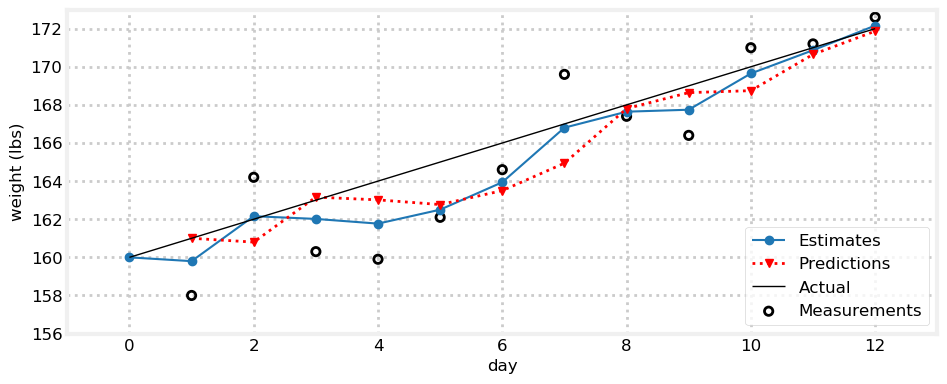That is pretty good! There is a lot of data here, so let's talk about how to interpret it. The thick blue line shows the estimate from the filter. It starts at day 0 with the initial guess of 160 lbs. The red line shows the prediction that is made from the previous day's weight. So, on day one the previous weight was 160 lbs, the weight gain is 1 lb, and so the first prediction is 161 lbs. The estimate on day one is then part way between the prediction and measurement at 159.8 lbs. Below the chart is a print out of the previous weight, predicted weight, and new estimate for each day. Finally, the thin black line shows the actual weight gain of the person being weighed.

Walk through this for each day, ensuring you understand how the prediction and estimates were formed at each step. Note how the estimate always falls between the measurement and prediction.

The estimates are not a straight line, but they are straighter than the measurements and somewhat close to the trend line we created. Also, it seems to get better over time.

The results of the filter may strike you as quite silly; of course the data will look good if we assume the conclusion, that our weight gain is around 1 lb/day! Let's see what the filter does if our initial guess is bad. Let's predict that there is a weight loss of 1 lb a day:

In :
e, p = predict_using_gain_guess(initial_estimate, -1.)
gh.plot_gh_results(weights, e, p, [160, 172])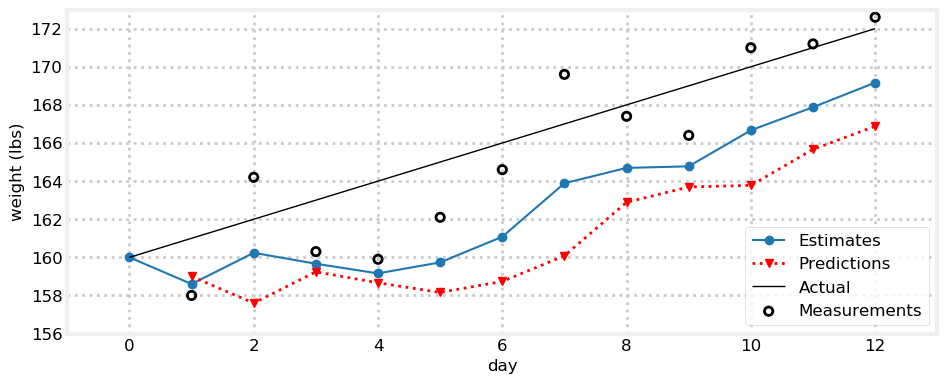That is not so impressive. The estimates quickly divert from the measurements. Clearly a filter that requires us to correctly guess a rate of change is not very useful. Even if our initial guess was correct, the filter will fail as soon as that rate of change changes. If I stop overeating the filter will have extreme difficulty in adjusting to that change. Note that it is adjusting! The estimates are climbing even though we tell it we are losing 1 lb a day. It just can't adjust fast enough.

But, 'what if'? What if instead of leaving the weight gain at the initial guess of 1 lb (or whatever), we compute it from the existing measurements and estimates. On day one our estimate for the weight is:

$$(160 + 1) + \frac{4}{10}(158-161) = 159.8$$

On the next day we measure 164.2, which implies a weight gain of 4.4 lbs (since 164.2 - 159.8 = 4.4), not 1. Can we use this information somehow? It seems plausible. After all, the weight measurement itself is based on a real world measurement of our weight, so there is useful information. Our estimate of our weight gain may not be perfect, but it is surely better than just guessing our gain is 1 lb. Data is better than a guess, even if it is noisy.

People really balk at this point, so make sure you are in agreement. Two noisy measurements of weight give us an implied weight gain/loss. That estimate is going to be very inaccurate if the measurements are inaccurate, but there is still information in this computation. Imagine weighing a cow with a scale accurate to 1 lb, and it shows that the cow gained 10 lbs. The cow might have gained 8 lbs up to 12 lbs, depending on the errors, but we know it gained weight, and roughly how much. This is information. What do we do with information? Never throw it away!

Back to my diet. Should we set the new gain/day to 4.4 lbs? Yesterday we thought the weight gain was 1 lb, today we think it is 4.4 lbs. We have two numbers, and want to combine them somehow. Hmm, sounds like our same problem again. Let's use our same tool, and the only tool we have so far - pick a value part way between the two. This time I will use another arbitrarily chosen number, $\frac{1}{3}$. The equation is identical as for the weight estimate except we have to incorporate time because this is a rate (gain/day):

$$\text{new gain} = \text{old gain} + \frac{1}{3}\frac{\text{measurement - predicted weight}}{1 \text{ day}}$$
In :
weight = 160.  # initial guess
gain_rate = -1.0  # initial guess

time_step = 1.
weight_scale = 4./10
gain_scale = 1./3
estimates = [weight]
predictions = []

for z in weights:
# prediction step
weight = weight + gain_rate*time_step
gain_rate = gain_rate
predictions.append(weight)

# update step
residual = z - weight

gain_rate = gain_rate + gain_scale   * (residual/time_step)
weight    = weight    + weight_scale * residual

estimates.append(weight)

gh.plot_gh_results(weights, estimates, predictions, [160, 172])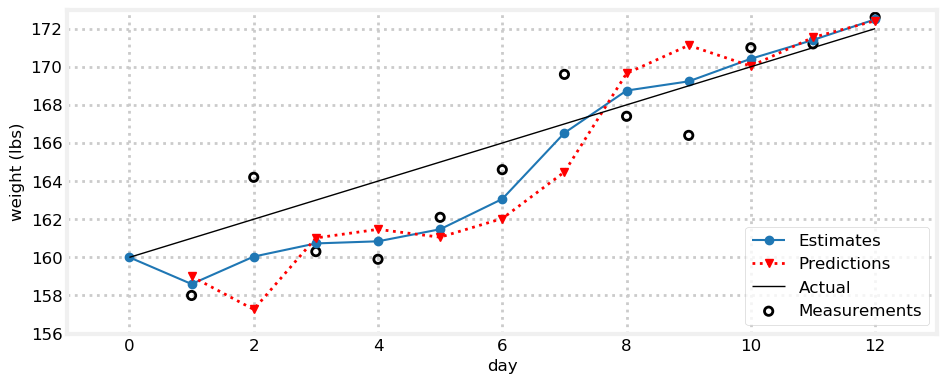I think this is starting to look really good. Because of the poor initial guess of the weight gain being -1 it takes the filter several days to accurately predict the weight, but once it does that it starts to accurately track the weight. We used no methodology for choosing our scaling factors of $\frac{4}{10}$ and $\frac{1}{3}$ (actually, they are poor choices for this problem), but otherwise all of the math followed from very reasonable assumptions. Recall that you can change the value of the parameter time_step to a larger value and re-run the cell if you want to see the plot drawn step-by-step.

One final point before we go on. In the prediction step I wrote the line

gain_rate = gain_rate


This obviously has no effect, and can be removed. I wrote this to emphasize that in the prediction step you need to predict the next value for all variables, both weight and gain_rate. This will become relevant shortly. In this case we are assuming that the gain does not vary, but when we generalize this algorithm we will remove that assumption.

## The g-h Filter¶

This algorithm is known as the g-h filter or the $\alpha$-$\beta$ filter. $g$ and $h$ refer to the two scaling factors that we used in our example. $g$ is the scaling we used for the measurement (weight in our example), and $h$ is the scaling for the change in measurement over time (lbs/day in our example). $\alpha$ and $\beta$ are just different names used for this factors.

This filter is the basis for a huge number of filters, including the Kalman filter. In other words, the Kalman filter is a form of the g-h filter, which I will prove later in the book. So is the Least Squares filter, which you may have heard of, and so is the Benedict-Bordner filter, which you probably have not. Each filter has a different way of assigning values to $g$ and $h$, but otherwise the algorithms are identical. For example, the Benedict-Bordner filter assigns a constant to $g$ and $h$, constrained to a certain range of values. Other filters such as the Kalman will vary $g$ and $h$ dynamically at each time step.

Let me repeat the key points as they are so important. If you do not understand these you will not understand the rest of the book. If you do understand them, then the rest of the book will unfold naturally for you as mathematical elaborations to various 'what if' questions we will ask about $g$ and $h$. The math may look profoundly different, but the algorithm will be exactly the same.

• Multiple data points are more accurate than one data point, so throw nothing away no matter how inaccurate it is.
• Always choose a number part way between two data points to create a more accurate estimate.
• Predict the next measurement and rate of change based on the current estimate and how much we think it will change.
• The new estimate is then chosen as part way between the prediction and next measurement scaled by how accurate each is.

Let's look at a visual depiction of the algorithm.

In :
book_plots.predict_update_chart()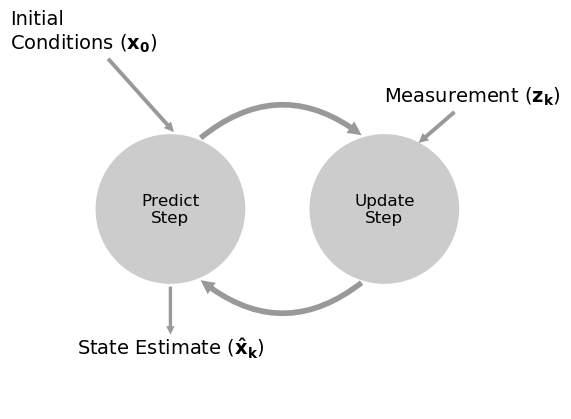Let me introduce some more formal terminology. The system is the object that we want to estimate. In this chapter the system is whatever we are trying to weigh. Some texts call this the plant. That terminology comes from control system theory. https://en.wikipedia.org/wiki/Plant_(control_theory)

The state of the system is the current configuration or values of that system that is of interest to us. We are interested only in the weight reading. If I put a 100 kg weight on the scale, the state is 100kg. We define the state based on what is relevant to us. The color of the scale is irrelevant to us so we do not include those values in the state. A QA engineer for the manufacturer might include color in the state so that she can track and control the manufacturing process.

The measurement is a measured value of the system. Measurements can be inaccurate, so it may not have the same value as the state.

The state estimate is our filter's estimate of the state. For example, for the 100 kg weight our estimate might be 99.327 kg due to sensor errors. This is commonly abbreviated to estimate, and I have done that in this chapter.

In other words, the state should be understood as the actual value of the system. This value is usually hidden to us. If I stepped on a scale you'd then have a measurement. We call this observable since you can directly observe this measurement. In contrast, you can never directly observe my weight, you can only measure it.

This language of hidden and observable is important. Any estimation problem consists of forming an estimate of a hidden state via observable measurements. If you read the literature these terms are used when defining a problem, so you need to be comfortable with them.

We use a process model to mathematically model the system. In this chapter our process model is the assumption that my weight today is yesterday's weight plus my weight gain for the last day. The process model does not model or otherwise account for the sensors. Another example would be a process model for an automobile. The process model might be "distance equals velocity times time. This model is not perfect as the velocity of a car can vary over a non-zero amount of time, the tires can slip on the road, and so on. The system error or process error is the error in this model. We never know this value exactly; if we did we could refine our model to have zero error. Some texts use plant model and plant error. You may also see system model. They all mean the same thing.

The predict step is known as system propagation. It uses the process model to form a new state estimate. Because of the process error this estimate is imperfect. Assuming we are tracking data over time, we say we propagate the state into the future. Some texts call this the evolution.

The update step is known as the measurement update. One iteration of the system propagation and measurement update is known as an epoch.

Now let's explore a few different problem domains to better understand this algorithm. Consider the problem of trying to track a train on a track. The track constrains the position of the train to a very specific region. Furthermore, trains are large and slow. It takes many minutes for them to slow down or speed up significantly. So, if I know that the train is at kilometer marker 23 km at time t and moving at 18 kph, I can be extremely confident in predicting its position at time t + 1 second. Why is that important? Suppose we can only measure its position with an accuracy of $\pm$ 250 meters. The train is moving at 18 kph, which is 5 meters per second. At t+1 seconds the train will be at 23.005 km yet the measurement could be anywhere from 22.755 km to 23.255 km. So if the next measurement says the position is at 23.4 we know that must be inaccurate. Even if at time t the engineer slammed on the brakes the train will still be very near to 23.005 km because a train cannot slow down very much in 1 second. If we were to design a filter for this problem (and we will a bit further in the chapter!) we would want to design a filter that gave a very high weighting to the prediction vs the measurement.

Now consider the problem of tracking a thrown ball. We know that a ballistic object moves in a parabola in a vacuum when in a gravitational field. But a ball thrown on Earth is influenced by air drag, so it does not travel in a perfect parabola. Baseball pitchers take advantage of this fact when they throw curve balls. Let's say that we are tracking the ball inside a stadium using computer vision, something I do at work. The accuracy of the computer vision tracking might be modest, but predicting the ball's future positions by assuming that it is moving on a parabola is not extremely accurate either. In this case we'd probably design a filter that gave roughly equal weight to the measurement and the prediction.

Now consider trying to track a helium party balloon in a hurricane. We have no legitimate model that would allow us to predict the balloon's behavior except over very brief time scales (we know the balloon cannot go 10 miles in 1 second, for example). In this case we would design a filter that emphasized the measurements over the predictions.

Most of this book is devoted to expressing the concerns in the last three paragraphs mathematically, which then allows us to find an optimal solution (in some mathematical sense). In this chapter we will merely be assigning different values to $g$ and $h$ in a more intuitive, and thus less optimal way. But the fundamental idea is to blend somewhat inaccurate measurements with somewhat inaccurate models of how the systems behaves to get a filtered estimate that is better than either information source by itself.

We can express this as an algorithm:

Initialization

1. Initialize the state of the filter
2. Initialize our belief in the state



Predict

1. Use system behavior to predict state at the next time step
2. Adjust belief to account for the uncertainty in prediction



Update

1. Get a measurement and associated belief about its accuracy
2. Compute residual between estimated state and measurement
3. New estimate is somewhere on the residual line



We will use this same algorithm throughout the book, albeit with some modifications.

## Notation¶

I'll begin to introduce the notations and variable names used in the literature. Some of this was already used in the above charts. Measurement is typically denoted $z$ and that is what we will use in this book (some literature uses $y$). Subscript $k$ indicates the time step, so $z_k$ is the data for this time step. A bold font denotes a vector or matrix. So far we have only considered having one sensor, and hence one sensor measurement, but in general we may have n sensors and n measurements. $\mathbf{x}$ denotes our state, and is bold to denote that it is a vector. For our scale example, it represents both the initial weight and initial weight gain rate, like so:

$$\mathbf{x} = \begin{bmatrix}x \\ \dot{x}\end{bmatrix}$$

Here I use Newton's notation of a dot over the x to denote velocity. More precisely, the dot implies the derivative of x with respect to time, which of course is the velocity. For a weight of 62 kg with a gain of 0.3 kg/day we have

$$\mathbf{x} = \begin{bmatrix}62 \\ 0.3\end{bmatrix}$$

So, the algorithm is simple. The state is initialized with $\mathbf{x_0}$, the initial estimate. We then enter a loop, predicting the state for time or step $k$ from the values from time (or step) $k-1$. We then get the measurement $z_k$ and choose some intermediate point between the measurements and prediction, creating the estimate $\mathbf{x}_k$.

## Exercise: Write Generic Algorithm¶

In the example above, I explicitly coded this to solve the weighing problem that we've been discussing throughout the chapter. For example, the variables are named "weight_scale", "gain", and so on. I did this to make the algorithm easy to follow - you can easily see that we correctly implemented each step. But, that is code written for exactly one problem, and the algorithm is the same for any problem. So let's rewrite the code to be generic - to work with any problem. Use this function signature:

def g_h_filter(data, x0, dx, g, h, dt):
"""
Performs g-h filter on 1 state variable with a fixed g and h.

'data' contains the data to be filtered.
'x0' is the initial value for our state variable
'dx' is the initial change rate for our state variable
'g' is the g-h's g scale factor
'h' is the g-h's h scale factor
'dt' is the length of the time step
"""


Return the data as a NumPy array, not a list. Test it by passing in the same weight data as before, plot the results, and visually determine that it works.

In :
from kf_book.gh_internal import plot_g_h_results
def g_h_filter(data, x0, dx, g, h, dt):

# uncomment to run the filter and plot the results
#book_plots.plot_track([0, 11], [160, 172], label='Actual weight')
#data = g_h_filter(data=weights, x0=160., dx=1., g=6./10, h=2./3, dt=1.)
#plot_g_h_results(weights, data)


### Solution and Discussion¶

In :
import matplotlib.pylab as pylab

def g_h_filter(data, x0, dx, g, h, dt=1.):
x_est = x0
results = []
for z in data:
# prediction step
x_pred = x_est + (dx*dt)
dx = dx

# update step
residual = z - x_pred
dx = dx + h * (residual) / dt
x_est = x_pred + g * residual
results.append(x_est)
return np.array(results)

book_plots.plot_track([0, 11], [160, 172], label='Actual weight')
data = g_h_filter(data=weights, x0=160., dx=1., g=6./10, h=2./3, dt=1.)
plot_g_h_results(weights, data)
print(weights)
print(data)

[158.0, 164.2, 160.3, 159.9, 162.1, 164.6, 169.6, 167.4, 166.4, 171.0, 171.2, 172.6]
[159.2   161.8   162.1   160.78  160.985 163.311 168.1   169.696
168.204 169.164 170.892 172.629]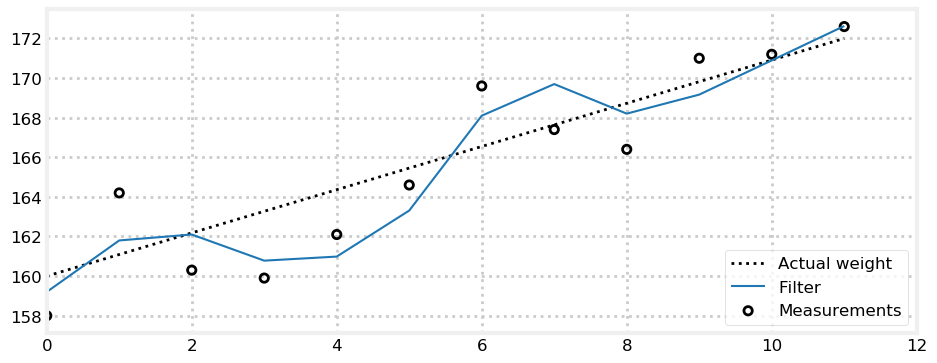This should have been straightforward. I just subtituted the variable names x0, dx, etc., for the variable names in the weight gain code. Nothing else needed to change.

## Choice of $g$ and $h$¶

The g-h filter is not one filter - it is a classification for a family of filters. Eli Brookner in Tracking and Kalman Filtering Made Easy lists 11, and I am sure there are more. Not only that, but each type of filter has numerous subtypes. Each filter is differentiated by how $g$ and $h$ are chosen. So there is no 'one size fits all' advice that I can give here. Some filters set $g$ and $h$ as constants, others vary them dynamically. The Kalman filter varies them dynamically at each step. Some filters allow $g$ and $h$ to take any value within a range, others constrain one to be dependent on the other by some function $f(\dot{}), \mbox{where }g = f(h)$.

The topic of this book is not the entire family of g-h filters; more importantly, we are interested in the Bayesian aspect of these filters, which I have not addressed yet. Therefore I will not cover selection of $g$ and $h$ in depth. Tracking and Kalman Filtering Made Easy is an excellent resource for that topic. If this strikes you as an odd position for me to take, recognize that the typical formulation of the Kalman filter does not use $g$ and $h$ at all. The Kalman filter is a g-h filter because it mathematically reduces to this algorithm. When we design the Kalman filter we use design criteria that can be mathematically reduced to $g$ and $h$, but the Kalman filter form is usually a much more powerful way to think about the problem. Don't worry if this is not too clear right now, it will clear once we develop the Kalman filter theory.

It is worth seeing how varying $g$ and $h$ affects the results, so we will work through some examples. This will give us strong insight into the fundamental strengths and limitations of this type of filter, and help us understand the behavior of the rather more sophisticated Kalman filter.

## Exercise: create measurement function¶

Now let's write a function that generates noisy data for us. In this book I model a noisy signal as the signal plus white noise. We've not yet covered the statistics to fully understand the definition of white noise. In essence, think of it as data that randomly varies higher and lower than the signal with no pattern. We say that it is a serially uncorrelated random variable with zero mean and finite variance. If you don't follow that, you will by the end of the Gaussians chapter. You may not be successful at this exercise if you have no knowledge of statistics. If so, just read the solution and discussion.

White noise can be generated by numpy.random.randn(). We want a function that we call with the starting value, the amount of change per step, the number of steps, and the amount of noise we want to add. It should return a list of the data. Test it by creating 30 points, filtering it with g_h_filter(), and plot the results with plot_g_h_results().

In :
# your code here


### Solution¶

In :
from numpy.random import randn
def gen_data(x0, dx, count, noise_factor):
return [x0 + dx*i + randn()*noise_factor for i in range(count)]

measurements = gen_data(0, 1, 30, 1)
data = g_h_filter(data=measurements, x0=0., dx=1., dt=1., g=.2, h=0.02)
plot_g_h_results(measurements, data)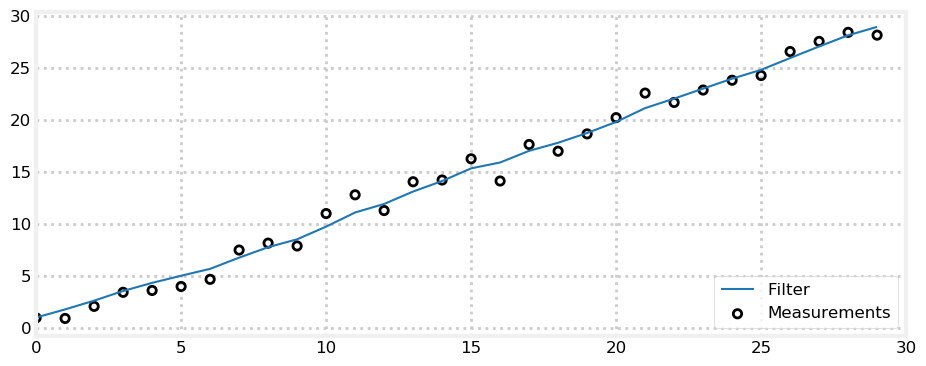### Discussion¶

randn() returns random numbers centered around 0 - it is just as likely to be greater than zero as under zero. It varies by one standard deviation - don't worry if you don't know what that means. I've plotted 3000 calls to randn() - you can see that the values are centered around zero and mostly range from a bit under -1 to a bit more than +1, though occasionally they are much larger.

In :
plt.plot([randn() for _ in range(3000)], lw=1);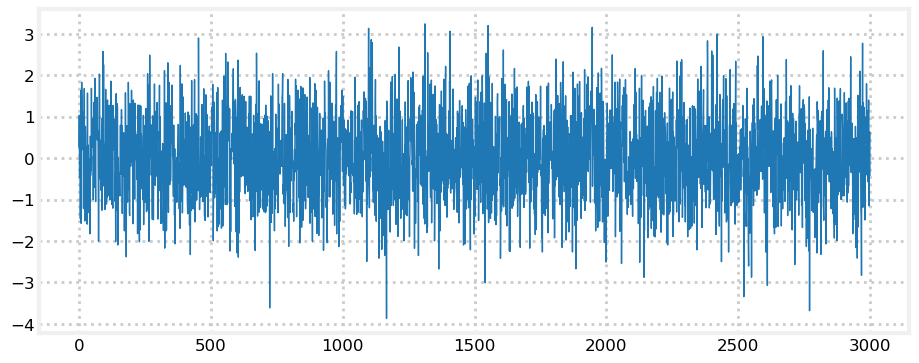Now write code that uses gen_data and g_h_filter to filter 100 data points that starts at 5, has a derivative of 2, a noise scaling factor of 10, and uses g=0.2 and h=0.02. Set your initial guess for x to be 100.

In :
# your code here


### Solution and Discussion¶

In :
zs = gen_data(x0=5., dx=2., count=100, noise_factor=10)
data = g_h_filter(data=zs, x0=100., dx=2., dt=1., g=0.2, h=0.02)
plot_g_h_results(measurements=zs, filtered_data=data)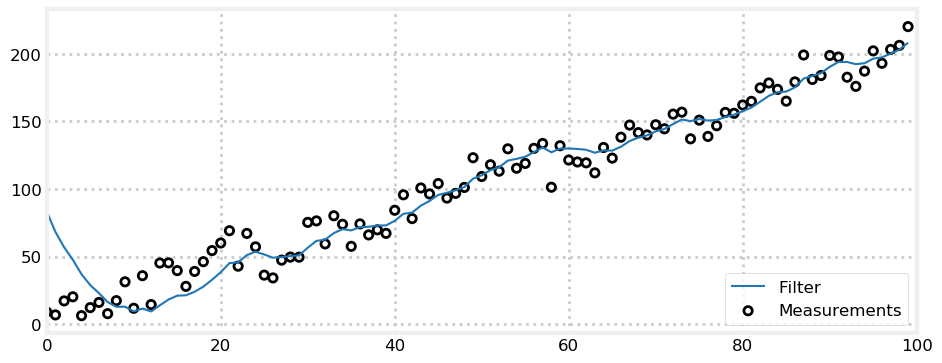The filter starts out with estimates that are far from the measured data due to the bad initial guess of 100. You can see that it 'rings' before settling in on the measured data. 'Ringing' means that the signal overshoots and undershoots the data in a sinusoidal type pattern. This is a very common phenomena in filters, and a lot of work in filter design is devoted to minimizing ringing. That is a topic that we are not yet prepared to address, but I wanted to show you the phenomenon.

## Exercise: Extreme Noise¶

Rerun the same test, but this time use a noise factor of 100. Remove the initial condition ringing by changing the initial condition from 100 down to 5.

In :
# your code here


### Solution and Discussion¶

In :
zs = gen_data(x0=5., dx=2., count=100, noise_factor=100)
data = g_h_filter(data=zs, x0=5., dx=2., g=0.2, h=0.02)
plot_g_h_results(measurements=zs, filtered_data=data)